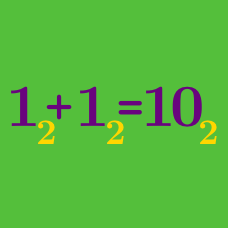Number Theory

# Number Bases

#### Challenge Quizzes

Express the decimal number $99$ as a hexadecimal (16-ary) number.

Note: $N_{16}$ means a hexadecimal number $N.$

If $X$ is a decimal number $181$ and $Y$ is a hexadecimal number $67_{16}$, what is the sum of the two numbers as a decimal number?

Note: $N_{16}$ means a hexadecimal number $N.$

If $X$ is a hexadecimal number $E2_{16}$ and $Y$ is a decimal number $181$, what is the sum of the two numbers as a hexadecimal number?

Note: $N_{16}$ means a hexadecimal number $N.$

Express a decimal number $53$ into a hexadecimal (16-ary) number.

Express the hexadecimal (16-ary) number $E7_{16}$ as a decimal number.

Note: $N_{16}$ means a hexadecimal number $N.$

×